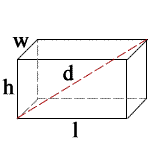## Rectangular Prism Calculator

 h = l = w = Units: d = 0 S = 0 V = 0

l = length, w = width, h = height, d = diagonal, S = surface area, V = volume

» Show Work

The rectangular prism has six faces that are rectangles, and has same cross-section.

### Rectangular prism FormulasVolume: V = lwh

Surface Area: S = 2(lw + lh + wh)

Space Diagonal: d = √(l2 + w2 + h2)

For example, when h = 2, l = 3, w = 4

1. To calculate d, use the following formula: √(l2 + w2 + h2). When you substitute l, w, and h for their values, you get √(32 + 42 + 22), which equals 5.38516 m.
2. To calculate S, use the following formula: 2(lw + lh + wh). When you substitute l, w, and h for their values, you get 2(3(4) + 3(2) + 4(2)), which equals 52 m2.
3. To calculate V, use the following formula: lwh. When you substitute l, w, and h for their values, you get 3(4)(2), which equals 24 m3.

Thinkcalculator.com provides you helpful and handy calculator resources.